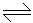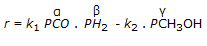# Chemical Engineering - Chemical Reaction Engineering

### Exercise :: Chemical Reaction Engineering - Section 7

46.

The ratio of moles of a reactant converted into the desired product to that converted into unwanted product is called

 A. operational yield B. relative yield C. selectivity D. none of these

Explanation:

No answer description available for this question. Let us discuss.

47.

The response curve for a step input signal from a reactor is called C-curve. The variance of C-curve in a 'tanks in series model' comprising of 'm' tanks is equal to

 A. m B. 1/m C. m D. m2

Explanation:

No answer description available for this question. Let us discuss.

48.

The eddy diffusivity for a liquid in plug flow must be

 A. 1 B. 0 C. ∞ D. between 0 and 1

Explanation:

No answer description available for this question. Let us discuss.

49.

For multiple reactions, the flow pattern within the vessel affects the

 A. size requirement B. distribution of reaction products C. both (a) and (b) D. neither (a) nor (b)

Explanation:

No answer description available for this question. Let us discuss.

50.

The rate expression for the gaseous phase reaction, CO + 2H2CH3OH, is given by,. Which of the following is not possible ?

 A. α = 1, β = 1, γ =1 B. α = 1, β = 2, γ = 1 C. α = 1/3, β = 2/3, γ = 1/3 D. α = 1/2, β = 1, γ = 1/2